5A: Pure Phases and their Transitions (Worksheet)

•• Contributed by Jim Clark
• Former Head of Chemistry and Head of Science at Truro School in Cornwall

Name: ______________________________

Section: _____________________________

Student ID#:__________________________

Work in groups on these problems. You should try to answer the questions without accessing the Internet.

Pure Phase Diagrams

A phase diagram is a type of chart used to show conditions (pressure, temperature, volume, etc.) at which thermodynamically distinct phases occur and coexist at equilibrium (at topic later on in the course).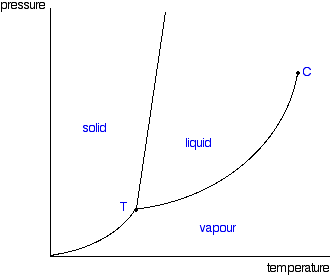Figure $$\PageIndex{1}$$: A typical phase diagram. These diagrams (including this one) are nearly always drawn highly distorted in order to see what is going on more easily. There are usually two major distortions. We'll discuss these when they become relevant.

If you look at Figure $$\PageIndex{1}$$, you will see that there are three lines, three areas marked "solid", "liquid" and "vapour", and two special points marked "C" and "T". Common components of a phase diagram are lines of equilibrium or phase boundaries, which refer to lines that mark conditions under which multiple phases can coexist at equilibrium. Phase transitions occur along lines of equilibrium.

The "C" point is known as the critical point. The temperature and pressure corresponding to this are known as the critical temperature and critical pressure. Point T on the diagram is called the triple point. If you think about the three lines which meet at that point, they represent the point that liquid, solid and gas coexist.

Constant Pressure Transitions

Suppose you had the following phase diagram for a simple pure substance: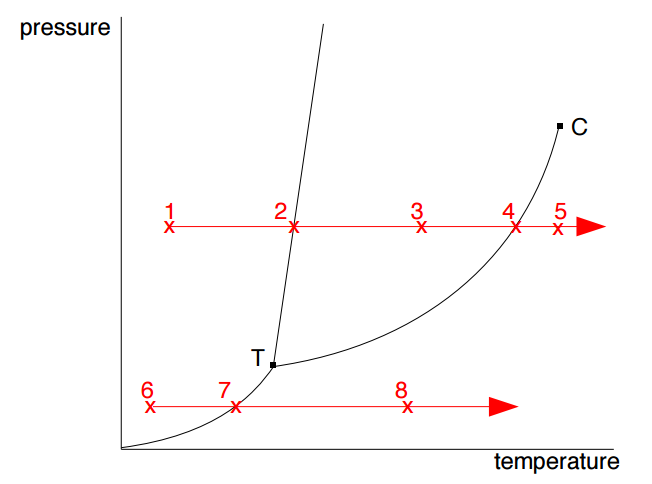1. What physical state would the substance be in under the conditions at point 1?
2. Suppose the temperature was then increased on this substance at constant pressure. What state(s) would the substance be in at each of points 2 to 5?
3. Suppose the constant pressure was 1 atmosphere. What useful information can you get from the diagram about the simple physical properties of the substance?
4. Suppose at a much lower pressure, the substance was under the conditions at point 6. Describe what happens to the substance as you increase the temperature from point 6 to point 8.

In a similar phase diagram: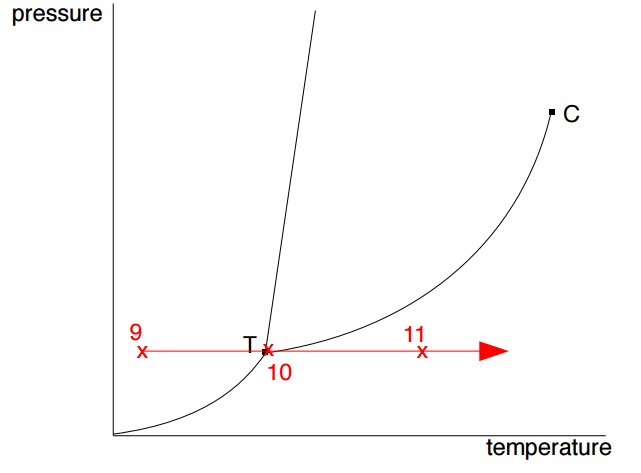1. What would be the state of the substance at point 9?
2. If the temperature was then increased at constant pressure, what state(s) would the substance be in at points 10, and 11?
3. Name, and explain the significance of, the point labeled T on these diagrams?

Constant Pressure Transitions

The next phase diagram looks at the effect of changing pressure at constant temperature.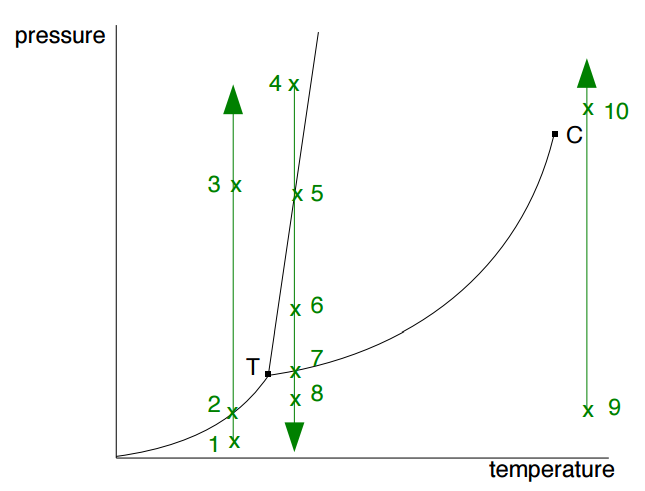1. Give the state(s) at each of the points 1 to 3.
2. Give the state(s) at each of the points 4 to 8.
3. Give the state(s) at points 9 and 10.
4. Name, and explain the significance of, the point C.

Curves of Equilibria

For the following two questions consider the two lines on the phase diagram below: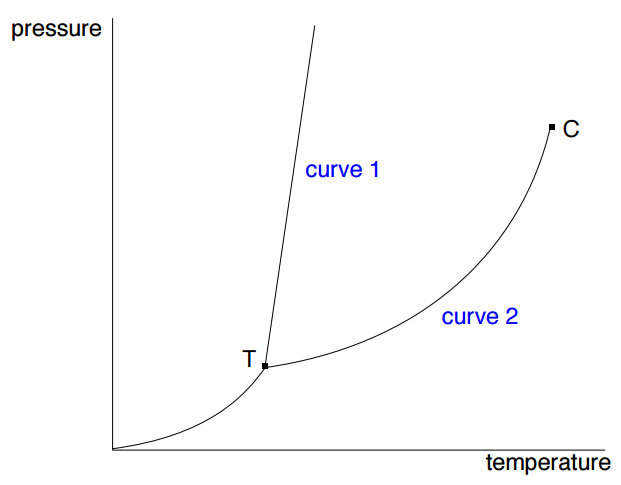1. Curve 1 can be thought of as showing the effect of pressure on melting point. Explain why most solids have a very steep curve, sloping slightly forwards as pressure increases.
2. Curve 2 can be thought of as showing the effect of pressure on boiling point. Explain why boiling point increases with pressure (this is a little hard at this stage, but give it a try).

Water

The next phase diagram is for water:1. Mark on the diagram the areas corresponding to ice, liquid water, and water vapor.
2. Explain what curve 1 represents, and why it slopes backwards unlike the corresponding curve 1 in the last diagram.

Carbon Dioxide

This is the phase diagram for carbon dioxide with some values marked on it:Solid carbon dioxide is commonly known as “dry ice”. Use the diagram above to explain how that term arises.